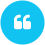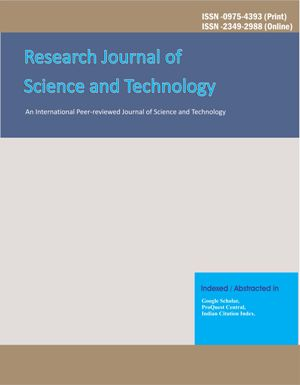ISSN

2349-2988 (Online)
0975-4393 (Print)

# FeketeSzego ̈ functional for a subclass of starlike functions with respect to symmetric points

Author(s): Harjinder Singh

Email(s):

DOI: Not Available

Department of Mathematics, Govt. Rajindra College, Bathinda (Punjab)
*Corresponding Author

ABSTRACT:
Let?? be the class of functionsf(z)=z+?_(n=2)^8¦?a_n z^n ?analytic in the unit discE={z:|z|<1}. Let S(a) denote the class of functions in ?? satisfying the condition Re{((1-a)zf^' (z))/(f(z)-f(-z) )+?a(zf^' (z) )?^'/(f(z)-f(-z) )^' }> 0,(0=a=1)and z?E. We are interested in determining the upper bounds of the FeketeSzego ¨ functional |a_3-µa_2^2 | for functions of the class S(a). MATHEMATICS SUBJECT CLASSIFICATION:30C45Harjinder Singh. FeketeSzego ̈ functional for a subclass of starlike functions with respect to symmetric points. Research J. Science and Tech 5(1): Jan.-Mar.2013 page 110-112.

## Recomonded Articles:Research Journal of Science and Technology (RJST) is an international, peer-reviewed journal, devoted to science and technology...... Read more >>>

RNI: Not Available
DOI: 10.5958/2349-2988